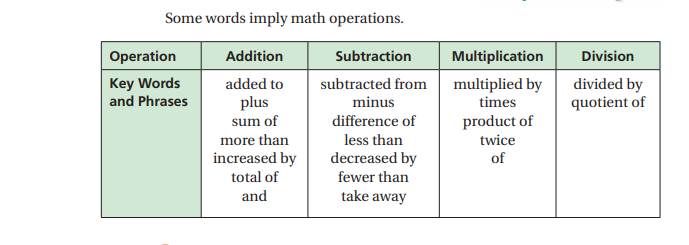## Current Math Topic

March: Your child is learning about Surface Area of 3-dimensional objects. Think of this a the surface that gets covered when you wrap a present! Kids learned about 3-D objects, studied various 3-D objects and learned to identify the number of faces each object has. Students made nets by cutting open 3-D objects and laying the faces flat to inspect each of the faces.

January - February: Your child is learning about Equations. An equation is a math sentence that contains: variables, constants, and coefficients and an equal sign.

This is the beginning of Algebra for your student! Kids are working with hands on equations, which are manipulatives that help them "balance" a written equation. Please ask your child to explain how hands on equations work!

December: Your child is learning about math properties. Properties to memorize are:

Commutative Property of Addition and Multiplication
Associative Property